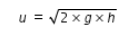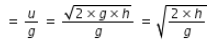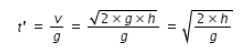# A stone thrown vertically upwards with initial velocity u reaches a height 'h'

A stone thrown vertically upwards with initial velocity u reaches a height ‘h’ before coming down.
Show that the time taken to go up is same as the time taken to come down.

we need to use the equations of motion,
v = u + a×t …(1)
v2= u2 + 2×a×S …(2)
where v is final velocity after time t, u is initial velocity, a is acceleration and S is distance traveled in time t.
Let u be the initial projection velocity of stone.
when the stone reaches the maximum height after time t, its final velocity is zero.
When the stone traveling vertically upwards, it is experiencing the retardation due to gravity.
hence we write the above equation of motion (1) as, 0 = u - g×t or t = u/g …(3)
eqn.(2) will be written as, 0 = u2 - 2×g×h, or…(4)
by substituting u from eqn.(4) in eqn.(3), we have t…(5)
After reaching maximum height, the stone descends with zero initial velocity,
accelerated downwards due to gravity and reaches the ground after time t’.
Eqns.(1) and (2) are written as follows for downward travel
v = 0+gt’ or t’ = v/g …(6)
v2 = 0+2gh or…(7)
from eqn.(4) and eqn.(7), we can conclude that initial projection velocity and
final velocity when the stone reaches the ground are equal
by substituting for v from eqn.(7) in eqn.(6), we get ,…(8)
from eqn.(5) and eqn.(8), time of ascending the height h is equal to time of descending from same height h